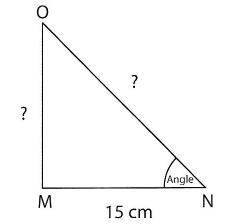Lesson 25 Triangle ratios

# Worksheet 2 Using triangle ratios

For these questions try to estimate or calculate the answer before drawing.

Do you need to draw any more triangles?

1. If you make the adjacent side 15c, what will be the length of the opposite side?

Can you explain what is happening?2. If you make the opposite side 6cm, what will be the length of the adjacent side?3. Do you have a general way of finding the missing side of a right-angled triangle

if you know one other angle and either the adjacent or the opposite side?